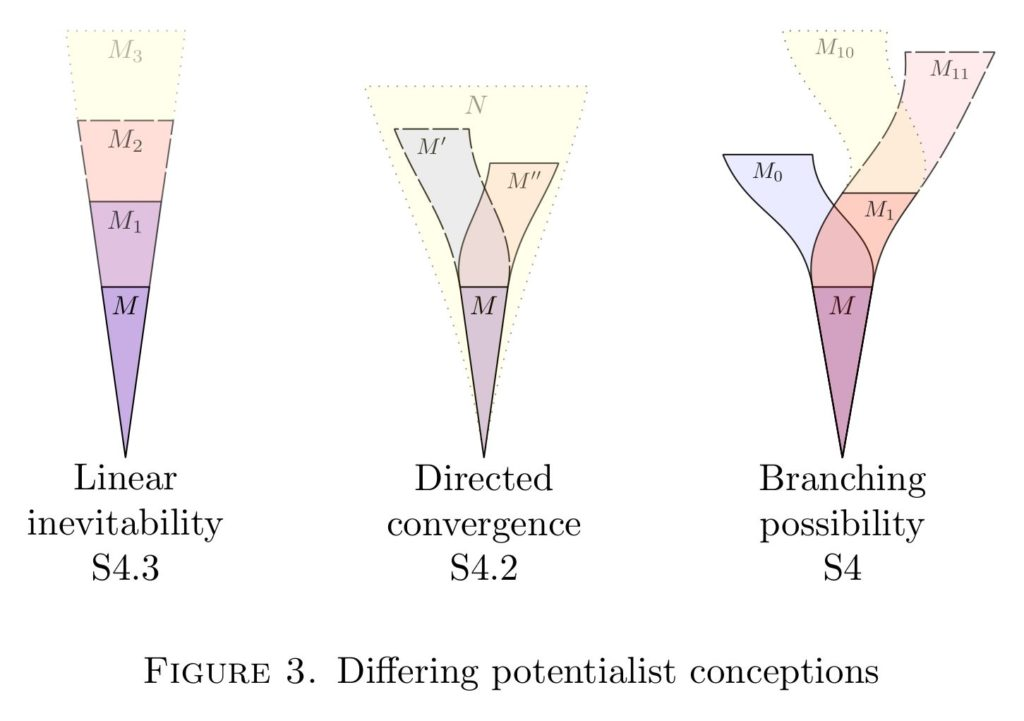# The modal logic of arithmetic potentialism and the universal algorithm

• J. D. Hamkins, “The modal logic of arithmetic potentialism and the universal algorithm,” ArXiv e-prints, pp. 1-35, 2018. (Under review)
@ARTICLE{Hamkins:The-modal-logic-of-arithmetic-potentialism,
author = {Joel David Hamkins},
title = {The modal logic of arithmetic potentialism and the universal algorithm},
journal = {ArXiv e-prints},
year = {2018},
volume = {},
number = {},
pages = {1--35},
month = {},
eprint = {1801.04599},
archivePrefix = {arXiv},
primaryClass = {math.LO},
note = {Under review},
url = {http://wp.me/p5M0LV-1Dh},
abstract = {},
keywords = {under-review},
source = {},
doi = {},
}Abstract. Natural potentialist systems arise from the models of arithmetic when they are considered under their various natural extension concepts, such as end-extensions, arbitrary extension, $\Sigma_n$-elementary extensions, conservative extensions and more. For these potentialist systems, I prove, a propositional modal assertion is valid in a model of arithmetic, with respect to assertions in the language of arithmetic with parameters, exactly when it is an assertion of S4. Meanwhile, with respect to sentences, the validities of a model are always between S4 and S5, and these bounds are sharp in that both endpoints are realized. The models validating exactly S5 are the models of the arithmetic maximality principle, which asserts that every possibly necessary statement is already true, and these models are equivalently characterized as those satisfying a maximal $\Sigma_1$ theory. The main proof makes fundamental use of the universal algorithm, of which this article provides a self-contained account.

In this article, I consider the models of arithmetic under various natural extension concepts, including end-extensions, arbitrary extensions, $\Sigma_n$-elementary extensions, conservative extensions and more. Each extension concept gives rise to an arithmetic potentialist system, a Kripke model of possible arithmetic worlds, and the main goal is to discover the modal validities of these systems.For most of the extension concepts, a modal assertion is valid with respect to assertions in the language of arithmetic, allowing parameters, exactly when it is an assertion of the modal theory S4. For sentences, however, the modal validities form a theory between S4 and S5, with both endpoints being realized. A model of arithmetic validates S5 with respect to sentences just in case it is a model of the arithmetic maximality principle, and these models are equivalently characterized as those realizing a maximal $\Sigma_1$ theory.

The main argument relies fundamentally on the universal algorithm, the theorem due to Woodin that there is a Turing machine program that can enumerate any finite sequence in the right model of arithmetic, and furthermore this model can be end-extended so as to realize any further extension of that sequence available in the model. In the paper, I give a self-contained account of this theorem using my simplified proof.The paper concludes with philosophical remarks on the nature of potentialism, including a discussion of how the linear inevitability form of potentialism is actually much closer to actualism than the more radical forms of potentialism, which exhibit branching possibility. I also propose to view the philosphy of ultrafinitism in modal terms as a form of potentialism, pushing the issue of branching possibility in ultrafinitism to the surface.

## 5 thoughts on “The modal logic of arithmetic potentialism and the universal algorithm”

1.none on said: